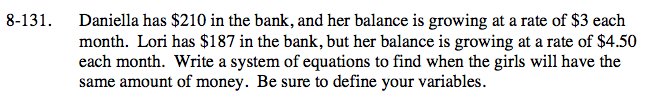Home > ACC7 > Chapter cc38 > Lesson cc38.3.1 > Problem8-131

8-131.

Daniella has $210 in the bank, and her balance is growing at a rate of$3 each month. Lori has $187 in the bank, but her balance is growing at a rate of$4.50 each month. Write a system of equations to find when the girls will have the same amount of money. Be sure to define your variables. Homework Help ✎Write two equations, one for Daniella and one for Lori,
where x = the number of months.

To find when the girls will have the same amount of money, set the two equations equal to each other.
210 + 3x = 187 + 4.5x

Daniella: y = 210 + 3x
Lori: y = 187 + 4.5x

$15\frac{1}{3}$

Be sure to know how to get this answer.